## Artigo

•Citado por SciELO
•Acessos

•Citado por Google
•Similares em SciELO
•Similares em Google

## versão impressa ISSN 0123-3033

### Ing. compet. vol.15 no.2 Cali jul./dez. 2013

On decidability properties of two fragments of the asynchronous π-calculus

Sobre la decidibilidad de dos fragmentos del p-cálculo asincrónico

Jesús A. Aranda B.
Escuela de Ingeniería de Sistemas y Computación, Universidad del Valle, Cali, Colombia
E-mail: jesus.aranda@correounivalle.edu.co

Eje temático: Systems engineering / Ingeniería de sistemas
Recibido: 27 de Abril de 2013
Aceptado: 09 de Septiembre de 2013

Abstract

In (Cacciagrano, et al., 2008) the authors studied the expressiveness of persistence in the asynchronous p-calculus, henceforth Ap. They considered Ap and three sub-languages of it, each capturing one source of persistence: the persistent-input calculus (PIAp), the persistent-output calculus (POAp), and the persistent calculus (PAp). They prove that, under some general conditions, there cannot be an encoding from Ap into a (semi)-persistent calculus preserving the must-testing semantics, a semantics sensitive to divergence.

In this paper we support and strengthen the separation results of (Cacciagrano, et al., 2008) by showing that convergence and divergence are two decidable properties in a fragment of POAp and PAp, in contrast to what happen in Ap. Thus, it is shown that there cannot be a (computable) encoding from Ap into PAp and in such a fragment of POAp, preserving divergence or convergence. These impossibility results don't presuppose any condition on the encodings and involve directly convergence for first time in the study of the expressiveness of persistence of Aπ

Keywords: Expressiveness, divergence, convergence, process calculi.

Resumen

En (Cacciagrano, et al., 2008) se estudió la expresividad de la persistencia en el p-cálculo asincrónico, Ap. En dicho artículo, los autores consideraron Ap y tres de sus fragmentos, cada uno de ellos capturando una fuente de persistencia: el fragmento con entradas persistentes (PIAp), el fragmento con salidas persistentes (POAp), y el fragmento con tanto entradas como salidas persistentes (PAp). Ellos demostraron que, bajo ciertas condiciones generales, no puede existir una codificación desde Ap en alguno de sus fragmentos preservando la semántica musttesting, una semántica sensible a la divergencia.

En este artículo se ratifican y fortalecen los resultados de separación de (Cacciagrano, et al., 2008) mostrando que tanto convergencia como divergencia son propiedades decidibles en un fragmento significativo de POAp y en PAp., a diferencia de lo que sucede en Ap. Así, se establece formalmente la no existencia de una codificación (decidable) de Ap en PAp o en el fragmento de POAp, preservando divergencia y convergencia. Estos resultados de separación no requieren de ninguna condición específica sobre las codificaciones e involucran directamente convergencia por primera vez en el estudio de la persistencia de Ap.

Palabras clave: Expresividad, divergencia, convergencia, cálculos de procesos.

1. Introduction

1.1 The π-calculus

The (polyadic) π-calculus (Milner, 1999) is one of the most influential formalisms for modeling and analyzing the behavior of concurrent systems; i.e. systems consisting of multiple computing agents, called processes that interact with each other. Indeed, the π-calculus has attained a wide range of applications in different areas of computer science and engineering, among others: Biology (Eccher, & Priami, 2006) business processes (Puhlmann, 2007), object-oriented programming (Jones, 1993), security (Abadi, & Gordon 1999), session types (Gay & Hole, 2005), and service oriented computing (Lucchi & Mazzara, 2007).

The relevance of this calculus has given rise to several variants; these variants can focus on some specific features or to be tailored to a specific domain. The exploration of the limitations, redundancies and capabilities of several variants is central in the expressiveness studies on process calculi.

1.2 Expressiveness

Most works on the expressiveness of the π-calculus consider questions such as whether a given variant can express certain behaviors, whether a given variant is as expressive as another one w.r.t. certain equivalence relation, or whether for some property, a given fragment is as hard as the full language.

Unfortunately, the subject of expressiveness in the π-calculus, and process calculi at large, is not a well-established discipline, or even a stable craft. Several guiding principles and cogent classification criteria have been put forth in several works such as (Palamidessi, 2003; Gorla, 2006; Vigliotti, et al., 2005; Gorla, 2008; Fu, & Lu, 2010). Hitherto, however, we do not have a general agreement as to what are the properties that a taxonomy of process calculi must consider in the way we have for the linguistic formalisms of computability, where the notion of language (generation) can be taken as the canonical measure for expressiveness. This is perhaps due to the great diversity of observations and properties often used to reason about concurrent behavior (e.g., divergence, convergence, failures, traces, barbs, must testing, bisimilarity, etc.). It may be the case that rather than being absolute, a taxonomy of concurrent calculi ought to be parametric on the observations we wish to make of processes. After all, concurrency is a field with a myriad of aspects for which we may require different terms of discussion and analysis.

The purpose of this paper is to show an expressiveness study of linearity and persistence Aπ features on the main variant of the π-calculus, , considering divergence and convergence as the properties of interest. Below, it is introduced the topic of this paper, some related works and our contributions.

1.3 Expressiveness issue: Linearity and persistence in the asynchronous π-calculus

In (Palamidessi, et al., 2006) the authors presented an expressiveness study of linearity and persistence of processes in the asynchronous version of the π-calculus, Aπ. Linearity (and persistence) is understood in a sense of that is similar to that used in (Girard 1987): the ability (incapability) of consuming a resource. The replication operator is central in (Palamidessi, et al., 2006) and plays a role similar to the "bang" operator from linear logic, also denoted as !.

The study in (Palamidessi, et al., 2006) is conducted in the asynchronous π - calculus, which naturally captures the notion of linearity and persistence also present in other calculi.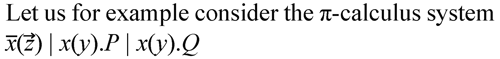This system represents a linear message with a datum z, tagged with x, that can be consumed by either (linear) receiver x(y).P or x(y).Q. Persistent messages (and receivers) can simply be specified using the replication operator that, as previously mentioned, creates an unbounded number of copies of a given process. One can then consider the existence of encodings from Aπinto three sub-languages of it, each capturing one source of persistence: the persistent-input calculus (PIAπ), defined as Aπ where inputs are replicated; the persistent-output calculus (POAπ), defined dually, i.e. outputs rather than inputs are replicated; the persistent calculus (PAπ), defined as Aπ but with all inputs and outputs replicated.

The main result in (Palamidessi, et al., 2006) basically states that we need one source of linearity, i.e. either on inputs (PIAπ) or outputs (POAπ) to encode the behavior of arbitrary Aπ processes via weak barbed congruence.

The notion of linearity (persistency) is present is several concurrency frameworks. Persistence of messages is present, e.g., in Concurrent Constraint Programming (CCP) (Saraswat, 1993), SPL (Crazzolara, & Winskel, 2001), and the Spi Calculus variants in (Amadio, et al., 200)). In all these formalisms messages cannot be consumed. In the π-calculus persistent receivers are used, for instance, to model functions, objects, higher order communications, or procedure definitions. Furthermore, persistence of both messages and receivers arise in the context of CCP with universally-quantified persistent ask operations (Fages, et al., 2001; Olarte, & Valencia 2008) and in the context of calculi for security, persistent receivers can be used to specify protocols where principals are willing to run an unbounded number of times (and persistent messages to model the fact that every message can be remembered by the spy).

Now, the previously mentioned positive result in (Palamidessi, et al., 2006) may give insights in the context of the expressiveness of the above frameworks. The main drawback of the work (Palamidessi, et al., 2006) is, however, that the notion of correctness for the encodings is based on weak barbed bisimulation (congruence), which is not sensitive to divergence. In particular, the encoding provided in (Palamidessi, et al., 2006) from into PIAπ is weak barbed congruent preserving but not divergence preserving. Although in some situations divergence may be ignored, in general it is an important issue to consider in the correctness of encodings (Gorla, 2006; Palamidessi, 2003; Cacciagrano, et al., 2006).

In (Cacciagrano, et al., 2008), the study of linearity and persistence complements the work (Palamidessi, et al., 2006) proving that, under some general conditions, there cannot be an encoding from into a (semi-) persistent calculus preserving the must-testing semantics; this semantics is sensible to divergence.

Unfortunately, in concurrency theory there is no a unified notion about what a good encoding is. There are several works about what properties should have an encoding (Palamidessi, 2003; Gorla, 2006; Vigliotti, et al., 2005; Gorla, 2008; Fu, & Lu, 2010; Nestmann, 2000). Considering this, it would be legitimate to ask, at what extent, the separation results from Aπ into the (semi-) persistent calculi depends on the general conditions in (Cacciagrano, et al., 2008) or only concerns divergence.

The por our main contribution is to show formally that two fragments of the (semi-) persistent subcalculi of Aπ are not as expressive as Aπ when divergence or convergences are considered. Unlike (Cacciagrano, et al., 2008), the separation results showed in this paper does not rely on some particular properties that an encoding should satisfy. The results are the following: Convergence and divergence are decidable in PAπ, Convergence and divergence are decidable in the fragment of POAπ where the replication operator is restricted to input processes and output processes.

As convergence and divergence are undecidable in , see Remark 1 in Section 3., then, there is no (computable) encoding from Aπ into these fragments.

The relevance of this paper does not rely only on the discriminatory results between different fragments of Aπ, but identifies fragments where it is possible to define a range of processes where it would be possible to determine automatically properties such as convergence and divergence. Decidability results for process calculi as those presented in this paper are important steps towards the development of formal verification tools for concurrent systems.

2. Preliminaries

This section introduces some notions, notations that will be used in the rest of the paper.

2.1 The asynchronous π-calculus: Aπ

Communication in the π-calculus is considered synchronous. The key property relies on the fact that the output and the input prefix impose a precedence over the terms which are underneath, such that once a communication involving the output and the input prefix occurs, the terms which were underneath the prefixes are unguarded at the same time. This behavior can be seen as a kind of acknowledgement of the execution of the communication over the processes involved in it.

Asynchronous π-calculus (Aπ) is a variant of the π-calculus introduced in (Boudol, 1992; Honda, & Tokoro, 1991). In this variant the communication can be seen as asynchronous, in the sense that the act of sending a datum and the act of receiving it can be seen as separate, hence not simultaneous. Aπ is obtained by restricting the term underneath the output prefix to be 0 (the null process). In this way the kind of acknowledgement provided by the precedence in the output prefix is lost. Moreover, an unguarded occurrence of x(y) can be thought of as a datum y in an implicit communication medium, tagged with x to indicate that it is available to any unguarded term of the form x(z).P. Thus, in the evolution of a term, the datum y can be considered to be sent when x(y) becomes unguarded, and to be received when x(y) disappears via an internal action.

2.1.1 Syntax

Names are the most primitive entities in the Aπ-calculus. We presuppose a countable set N of (port, links or channel) names, ranged over by , y,... . For each name x, we assume a co-name x thought of as complementary, so we decree that x= x. The other entity in the π-calculus is a process. Processes are built from names as follows.

Definition 1 (Syntax) Processes in Aπ-calculus are given respectively by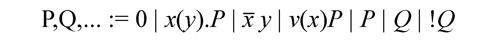The process (summation) 0 does nothing. xy and x(y).P represent the output and input process respectively, xy is a process which can output a datum y on channel x. x(y).P is a process which can perform an input action on channel x and then it behaves like P{z/y}, the process which has replaced every occurrence of the name y, by the datum z received. {z/y} is a substitution of z by y, x(y) is called a guard or (input) prefix. In P | Q, the parallel composition of P and Q, P and Q can proceed independently or can synchronize via shared names. In (ν x)P , the name x is declared private to P , i.e. initially, components of P can use x to interact with one another but not with other processes, the scope of x could change as a result of interaction between processes as will be seen later. Finally, the replication !P can be thought of as unboundedly many P's in parallel P | P | P | ..., replication is the means to express infinite behaviour.

In each of x(y).P and (v y)P, the occurrence of y is bound with scope P . An occurrence of a name in a process is bound if it is under the scope of a binding occurrence of the name. An occurrence of a name is free if it is not bound. Given Q we define its bound names bn(Q) as the set of names with a bound occurrence in Q, and its free names fn(Q) as the set of names with a non-bound occurrence in Q, hence n(Q) = fn(Q) ∪ bn(Q) is the set of names of Q.

In this paper, we consider a version of Aπ without τ and choice as proposed in (Boudol, 1992; Honda, & Tokoro, 1991).

2.1.2 Semantics

The semantics of the language described above is made precise by a labeled transition system. A αtransition P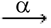Q says that P can perform an action α and evolve into Q. The set of actions used in the transition system is composed by x y, x y, x(y), t. x y, a free output, sends the name y on the name x, x y , an input, receives the name y on the name x, x(y), a bound output, sends a fresh name on x and t is an internal action .

Definition 2 (Semantics) The labeled transition relation αis given by the rules in Table 1. Omitted from Table 1 are the symmetric forms of Par-L, Com- L and Close-L.

2.2 Divergence and convergence

Definition 3 We say that P is stable iff P cannot perform any action α.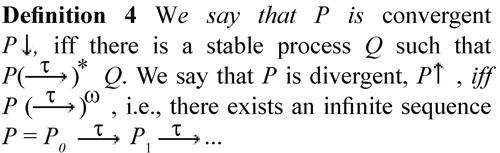2.3 Semi-persistence in Aπ

Here we define the syntactic restrictions of Aπ that are considered in this paper.

The fragment of persistent-output calculus POAπri, arises as from Aπ by requiring all outputs to be replicated where the replication operator is restricted to input processes and output processes.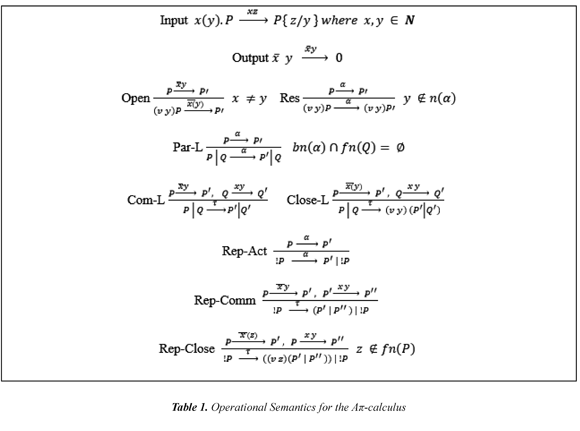Processes in POAπri are generated by the following grammar:

P,Q,... := 0 | x(y).P | !x (y).P | !x y | (vx)P | (P | Q )

Finally, we have the persistent calculus π, a subset of Aπ where output and input processes must be replicated. Processes in PAπ are generated by the following grammar:

P,Q,... := 0 | !x (y).P | !x y | (vx)P | (P | Q ) | !P

The relationfor POAπri and PAπ can be equivalently defined as in Table 1, with Output replaced with Output(POAπri), and Input and Output replaced with Input(PAπ) and Output(PAπ) rules showed below. The new rules reflect the persistent-output nature of POAπri (Rule Output(POAπri)), and the persistent nature of PAπ (Rules Input and Output(PAπ)). Notice that these new rules can be derived directly from the application of the Rules Input, Output, and Rep-Act in Table 1.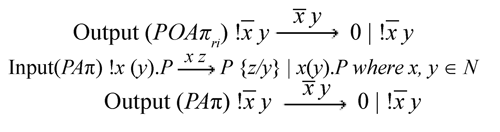Remark 1 Notice that, unlike to the presentation of Aπ, its syntactic restrictions does not include terms as !P explicitly. However, it is clear that all the terms from these restricted variants correspond to Aπ terms. Considering this, and for the sake of uniformity in the presentation of the proofs, we consider processes in Lemmas 1, 2, 3, and 4 as Aπ terms rather than restricted language terms. Clearly the results obtained from these lemmas are valid for the two syntactic variants.

3. Decidability results for POAπri and PAπ

We shall prove that there is no computable encoding preserving divergence or convergence from into POAπri and PAπ. We do this by proving that unlike for , divergence and convergence are decidable for these POAπri and PAπ processes.

Below, a remark on the undecidabiliy of convergence and divergence in .

Remark 2 Convergence and divergence are undecidable in . In (Busi, et al, 2009), it was proved the undecidability of convergence and divergence for the calculus CCS. That result is extended directly to by using the encoding from π-calculus with recursive functions into replication showed in (Sangiorgi, Walker, 2001). The encoding from guarded-choice π-calculus into choice-free π-calculus given in (Palamidessi, et al, 2006), and either Honda and Tokoro's encoding or Boudol's encoding from π-calculus into proposed in (Boudol, 1992) and (Honda, & Tokoro, 1991). All of these encodings preserve and reflect divergence and convergence.

We need to prove that the set of reachable processes through a τ-action can be computed; Succ(P) = {P' P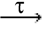, P'} is computable.

Without lose of generality, we assume that all the bound and free names are distinct in every process we consider in this section. Notice that every process can be transformed into an equivalent process with distinct names by using α-conversion (Sangiorgi, & Walker, 2001).

It is well known that the relationis image finite (Sangiorgi, & Walker, 2001). Therefore the set of successors of a process P, Succ(P), is finite. Here we describe how to build this set.

3.1 Computing successors

Now, it will be illustrated how to calculate derivative processes fron any Aπ process P is computable. The approach is to show a function doing the corresponding calculations. Depending on the nature of the derivation, one of the following lemmas is the most suitable. The computability of the derivative processes is extended trivially to the terms defined in its syntactic restrictions of Aπ, i.e POAπriand PAπ.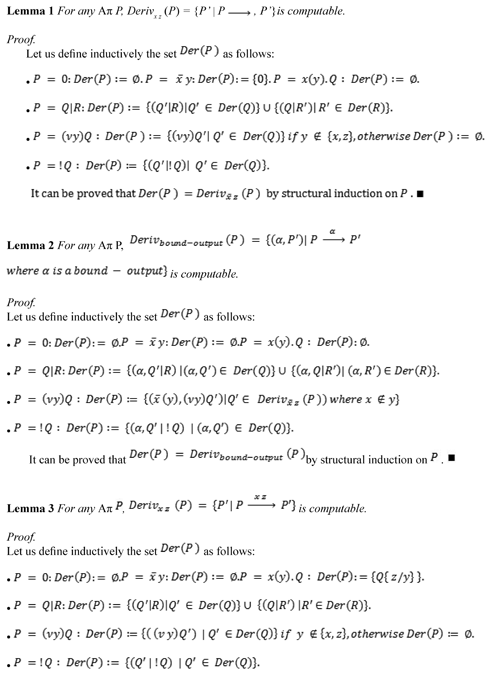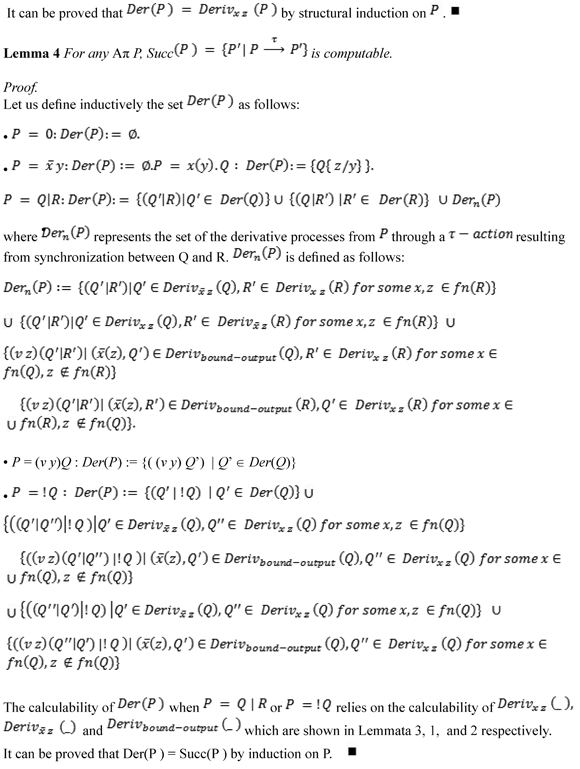3.2 Decidability of convergence and divergence

Now, we can show that convergence and divergence are decidable for POAπri and PAπ. The next theorem shows the decidability of divergence and convergence in PAπ.

Theorem 1 Divergence and convergence are decidable in PAπ. Proof.

From the persistent nature of both input and output prefixes in PAπ, we know that if a synchronisation happens in a POAπri process then there must be an infinite τ-labelled computation from such a PAπ process. Hence a PAπ process P is divergent if and only if Succ(P)>0 and we can say that a PAπ process P is convergent if and only if Succ(P)=0.■

Now, we consider the decidability of convergence and divergence for POAπri First we need to introduce the notion of occurrence of linear input prefix. A linear input prefix is the input prefix that is not under the scope of the replication operator.

Definition 6(Occurrences of linear input prefix) Let PPOAπri.The maximal number of occurrences of linear inputs in P,LinearInp(P)is given inductively as follows: LinearInp(0)=0, LinearInp(!x-)=0, LinearInp (a( x ). P )=1+ LinearInp ( P ), LinearInp(!P)=0, LinearInp((vx)P)=LinearInp(P), LinearInp(P | Q)==LinearInp(P)+=LinearInp(Q).

The following proposition says that only input actions that come from linear input prefixes can participate, by synchronization, in a finite maximal sequence of τ-actions.

Proposition 1 Let P, P´ POAπri such that PP´ and P´ is convergent. Then P is convergent and each τ -move from P into P´ is produced by a synchronization between an output and an input action coming from a linear input prefix.

Proof.

To prove the first part, to obtain a contradiction, let us suppose that P is non-convergent, i.e. there is no maximal finite computation from P. As any maximal computation from can be seen as the ending part of a maximal computation from P passing through. Each maximal computation from must be infinite. Therefore. is nonconvergent, a contradiction.

To prove the second part, to obtain a contradiction, let us suppose that there is a τ -move from P into (convergent) produced by a synchronisation between an output and an input action coming from an input prefix being under the scope of a replication operator. Since output actions are persistent at time in POAπri, i.e. once an output action can be performed, the same action can be executed at anytime later on, and the input actions coming from input prefix being under the scope of a replication operator are persistent as well, there is possible to perform a synchronization from and any of its τ-derivative processes. Hence cannot be convergent, a contradiction. ■

As a corollary from Proposition 1, we have the following proposition:

Proposition 2 Let P, P´ POAπri such that τ such that PP´ and P´ is convergent. Then LinearInp(P) ≥ 1.

A crucial observation to prove the decidability of convergence and divergence in POAπri is that the number of occurrences of linear input prefix decreases as long as a finite computation is performed.

Proposition 3 Let P, P´ POAπri such that such that PP´ and P´ is convergent. Then LinearInp(P) = LinearInp(P) - 1.

Proof.

From Proposition 1, we know that any τ-move from P intocorresponds to a synchronisation where the input action comes from a linear input prefix. The participation of this kind of input action implies that an occurrence of a linear input prefix is consumed from P. Notice that although the execution of this input action can substitute names in P, the linear or persistent nature of the rest of the process remains unchanged. As for the output action, the consumption of an output action does not alter the number of occurrences of linear input prefix; it is due to the asynchronous nature of the calculus.■

The following Lemma gives an upper bound of the length of the maximal finite computations that depends on the number of occurrences of linear inputs prefix. Notice that the lower bound does not depend on this number, e.g. a(x).0 | a(x).0 |...| a(x).0 is stable.

Lemma 5 Let P POAπri . For each maximalfinite τ-labeled computation c from P, length(c) LinearInp(P).

Proof.

Let us consider any maximal finite computation from P: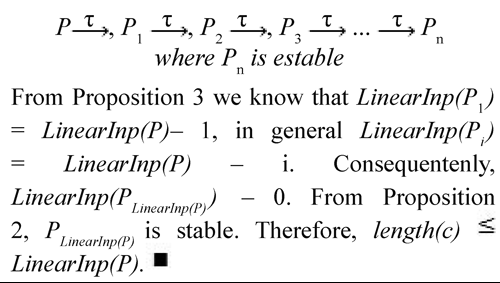From the computable function Succ, we can define and calculate a function Succi (P)={P | P'P' ' for some P Succi-1(P)} where Succ1(P) = Succ(P) and Succ0(P) = {P}, in a similar way we can identify the stable processes derivable from P at i τ-actions by the function SuccSt i (P) ={P' ' | P'P' ' for some P' Succi-1(P) and ∈ Succ(P' ' ) ={}}.

Now, it is easy to see from Lemma 5 and by using the functionSucci and SuccSti, which are computable from Lemma 4, that divergence and convergence are decidable.

Theorem 2 Divergence is decidable in POAπri . Proof. From Lemma 5, a POAπri process P is divergent if and only if there is at least one computation from P whose length is greater than LinearInp(P). It can be checked whether such a computation exists by ver- ifying that | SuccSt LinearInp(P)+1 (P)| ≥ 0. From Lemma 4 it is clear that SuccSt LinearInp(P)+1 (P) can be straightforwardly calculated.

Theorem 3 Convergence is decidable in POAπri . Proof. From Lemma 5, a POAπri process P is convergent if and only if there is at least one maximal computation from P whose length is less or equal to LinearInp(P), i.e. if there is at least one stable process derivable from P at most in LinearInp(P) τ-moves. It can be checked whether such a stable process exists by verifying that |SuccSt 0 (P) | + |SuccSt 1 (P)| + | SuccSt 2 (P)| + . . . + | SuccSt LinearInp(P) (P )| ≥ 1. From Lemma 4 it is clear that SuccSt i (P) for any natural number i can be straightforwardly calculated.

As corollary from Theorem 2 and Theorem 3 and considering Remark 5.1.1 we obtain the following separation result:

Theorem 4 There is no encoding preserving and reflecting divergence (convergence) from Aπ into POAπri.

4. Conclusions

In this paper we studied the decidability of divergence and convergence in two fragments of : POAπri and PAπ. As main contribution we showed that these two properties are decidable in both fragments.

As the divergence-sensitive nature of Testing Semantics (Nicola, & Hennessy, 1984) and the failures-sensitive nature of Failures Semantics (Milner, 1989), these results support and strengthen the separation results from (Cacciagrano, et al., 2008). This paper does not take into account particular properties that an encoding should satisfy, in addition this paper extends the expressiveness gap considering for first time convergence.

Notice that although the results for PAπ could be obtained straightforwardly from the results for POAπri , the nature of the separation is different in both cases: the separation results for PAπ seems to rely on the persistence nature of the processes exclusively, thus, we claim that this result is valid for the full synchronous π-calculus, as the results of (Cacciagrano, et al., 2008). On the other hand, the expressiveness gap between Aπ intoPOAπri relies on asynchrony, and then this result seems to be specialized for this fragment.

Although it seems that the results from this paper are not unexpected, one of the most relevant and important contributions of this paper relies on identifying precisely a range of processes where it would be possible to determine automatically properties such as convergence and divergence. A process calculi term can be used to model several concurrent real-life systems. Decidability results for process calculi are an important contribution towards the development of formal verification tools for concurrent systems.

Although this paper shows strong impossibility results on two fragments, it is necessary to extend this work and to explore these properties on full POAπ and PIAπ. In a near future, we expect to analyze how to model a Turing-equivalent model preserving divergence (convergence) into PIAπ, as some evidence (Aranda, 2009) suggests that PIAπ is more expressive than POAπ.

5. References

Abadi, M., & Gordon, A. (1999). A calculus for cryptographic protocols: The spi calculus. Inf. Comput , 148 (1), 1-70.         [ Links ]

Alves-Foss, J. (2000). An efficient secure authenticated group key exchange algo- rithm for large and dynamic groups. In Proceedings of the 23rd National Information Systems Security Conference (pp. 254-266). Recovered 2015/01/20 http://citeseerx.ist.psu.edu/viewdoc/download?doi=10.1.1.5.6947&rep=rep1&type=pdf        [ Links ]

Amadio, R., Lugiez, D., & Vanackere,. V. (2003). On the symbolic reduction of processes with cryptographic functions. Theor. Comput. Sci. , 290 (1), 695-740.         [ Links ]

Aranda, J. (2009). On the expressivity of Infinite and local behaviour in fragments of the II-calculus . Tesis de Doctorado, Escuela de Ingeniería de Sistemas y Computación, Universidad del Valle, Cali, Colombia.         [ Links ]

Aranda, J., Di Giusto, C., Nielsen, M., & Valencia, F. (2007). CCS with replication in the Chomsky hierarchy: the expressive power of divergence. In Z. Shao (Ed.). Lecture Notes in Computer Science (vol. 4807) (pp. 383-398). New York: Springer.         [ Links ]

Aranda, J., Valencia, F., & Versari, C. (2009). On the expressive power of restriction and priorities in ccs with replication. In L. de Alfaro (Ed.). Lecture Notes in Computer Science (vol. 5504) (pp. 242-256). New York: Springer.         [ Links ]

Borger, E., Gradel, E., & Gurevich, Y. (1997). The Classical Decision Problem . Berlin: Springer Verlag.         [ Links ]

Boudol, G. (1992). Asynchrony and the pi-calculus . Technical Report RR-1702. Antipolis: INRIA Sophia.         [ Links ]

Busi, N., Gabbrielli, M., & Zavattaro, G. (2009). On the expressive power of recursion, replication and iteration in process calculi. Mathematical Structures in Computer Science , 19 (6), 1223­1263.         [ Links ]

Busi, N. (2002) Analysis issues in petri nets with inhibitor arcs. Theor. Comput. Sci , 275 (1-2), 127-177.         [ Links ]

Busi, N., Gabbrielli, M., & Zavattaro, G. (2003) Replication vs. recursive definitions in channel based calculi. Lecture Notes in Computer Science 2719, 133-144.         [ Links ]

Busi, N., Gabbrielli, M., & Zavattaro, G. (2004) Comparing recursion,replication, and iteration in process calculi. Lecture Notes in Computer Science (vol. 3142) (pp. 307-319). New York: Springer.         [ Links ]

Cacciagrano, D., & Corradini, F. (2001). On synchronous and asynchronous communication paradigms. Lecture Notes in Computer Science (vol. 2202) (pp. 256-268). New York: Springer.         [ Links ]

Cacciagrano, D., Corradini, F., Aranda, J., & Valencia, F. (2008). Linearity, persistence and testing semantics in the asynchronous pi-calculus. Electr. Notes Theor. Comput. Sci. , 194 (2), 59-84.         [ Links ]

Cacciagrano, D., Corradini, F., & Palamidessi, C. (2006) Separation of synchronous and asynchronous communication via testing. Electr. Notes Theor. Comput. Sci , 154 (3), 95-108.         [ Links ]

Crazzolara, F., & Winskel, G. (2001). Events in security protocols. In ACM. ACM Conference on Computer and Communications Security (pp. 96-105). New York: ACM.         [ Links ]

Eccher, C., & Priami, C. (2006). Design and implementation of a tool for translating sbml into the biochemical stochastic pi-calculus. Bioinformatics , 22 (24), 3075-3081.         [ Links ]

Fages, F., & Ruet, P., & Soliman, S. (2001). Linear Concurrent Constraint Programming: Operational and Phase Semantics. Inf. Comput , 165(1), 14-41.         [ Links ]

Fu, Y., & Lu, H. (2010). On the expressiveness of interaction. Theoretical Computer Science , 411 (11-13), 1387-1451.         [ Links ]

Gay, S., & Hole, M. (2005). Subtyping for session types in the pi calculus. Acta Inf , 42 (2-3), 191-225.         [ Links ]

Gorla, D. (2006). On the relative expressive power of asynchronous communication primitives. Lecture Notes in Computer Science (vol. 3921) (pp. 47-62). New York: Springer.         [ Links ]

Gorla, D. (2008). Towards a unified approach to encodability and separation results for process calculi. Lecture Notes in Computer Science (vol. 5201) (pp. 492-507). New York: Springer.         [ Links ]

Honda, K. & Tokoro, M. (1991). An object calculus for asynchronous communication . Lecture Notes in Computer Science (vol. 512) (pp. 133-147). New York: Springer.         [ Links ]

Jones, C. (1993). A pi-calculus semantics for an object-based design notation. Lecture Notes in Computer Science (vol. 715) (pp. 158-172). New York: Springer.         [ Links ]

Lucchi, R., & Mazzara, M. (2007). A p-calculus based semantics for ws-bpel. J. Log. Algebr. Program , 70 (1), 96-118.         [ Links ]

Milner, R., (1989). Communication and Concurrency . New York: Prentice Hall.         [ Links ]

Milner, R., (1999). Communicating and Mobile Systems: the p-calculus . Cambridge (MA): Cambridge University Press.         [ Links ]

Nestmann, U., (2000). What is a "Good" Encoding of Guarded Choice? Inf, Comput , 156 (1-2). 287-319.         [ Links ]

Nicola, R., & Hennessy, M. (1984). Testing equivalences for processes. Theor. Comput. Sci. , 34, 83-133.         [ Links ]

Olarte, C., & Valencia, F. (2008). The expressivity of universal timed CCP: undecidability of Monadic FLTL and closure operators for security. In ACM. Proceedings of the 10th international ACM SIGPLAN conference on Principles and practice of declarative programming (pp. 8-19). New York: ACM.         [ Links ]

Palamidessi, C. (2003). Comparing the expressive power of the synchronous and asynchronous pi­calculi. Mathematical Structures in Computer Science , 13 (5), 685-719.         [ Links ]

Palamidessi, C., Saraswat, V., Valencia, F., & Victor, B. (2006). On the expressiveness of linearity vs persistence in the asychronous pi­calculus. In IEEE. Logic in Computer Science, 2006 21st Annual IEEE Symposium on (pp. 59 - 68). Seattle (WA): IEEE.         [ Links ]

Phillips, I. (2008). CCS with priority guards. J. Log. Algebr. Program , 75 (1), 139- 165.         [ Links ]

Puhlmann, F. (2007). Soundness verification of business processes specified in the pi-calculus. Lecture Notes in Computer Science (vol. 4803) (pp. 6-23). New York: Springer.         [ Links ]

Saraswat, V. (1993). Concurrent Constraint Programming . Cambridge (MA): The MIT Press.         [ Links ]

Vigliotti, M., Phillips, I. & Palamidessi, C. (2005). Separation results via leader election problems. Lecture Notes in Computer Science (vol. 4111) (pp. 172-194). New York: Springer.         [ Links ]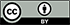Revista Ingeniería y Competitividad por Universidad del Valle se encuentra bajo una licencia Creative Commons Reconocimiento - Debe reconocer adecuadamente la autoría, proporcionar un enlace a la licencia e indicar si se han realizado cambios. Puede hacerlo de cualquier manera razonable, pero no de una manera que sugiera que tiene el apoyo del licenciador o lo recibe por el uso que hace.